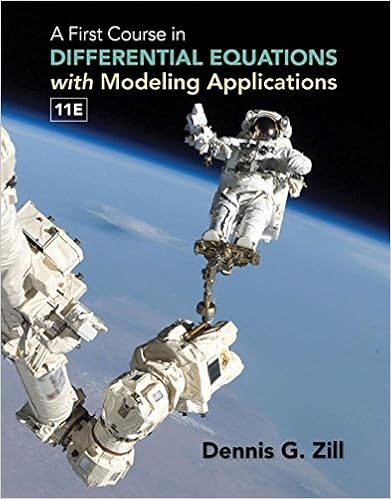# Solution using eq 4 with f x 1 x 2 a n 1 n 2 and m

• Assignment
• 202
• 100% (3) 3 out of 3 people found this document helpful

This preview shows page 76 - 78 out of 202 pages.

##### We have textbook solutions for you!
The document you are viewing contains questions related to this textbook.The document you are viewing contains questions related to this textbook.
Chapter 6 / Exercise 3
A First Course in Differential Equations with Modeling Applications
ZillExpert Verified
solution Using Eq. (4) with f (x) = 1 x 2 , a n = 1 n 2 and M = 40000, we find S 40000 + 40001 dx x 2 n = 1 1 n 2 S 40001 + 40001 dx x 2 . Now, S 40000 = 1 . 6449090672 ; S 40001 = S 40000 + 1 40001 = 1 . 6449090678 ; and 40001 dx x 2 = lim R →∞ R 40001 dx x 2 = − lim R →∞ 1 R 1 40001 = 1 40001 = 0 . 0000249994 . Thus, 1 . 6449090672 + 0 . 0000249994 n = 1 1 n 2 1 . 6449090678 + 0 . 0000249994 , or 1 . 6449340665 n = 1 1 n 2 1 . 6449340672 . Since π 2 6 1 . 6449340668, our approximation is consistent with Euler’s result. 88. Using a CAS and Eq. (5) from Exercise 85, determine the value of n = 1 n 6 to within an error less than 10 4 . Check that your result is consistent with that of Euler, who proved that the sum is equal to π 6 / 945. solution According to Eq. (5), if we choose M so that (M + 1 ) 6 < 10 4 , we can then approximate the sum to within 10 4 . Solving (M + 1 ) 6 = 10 4 gives M + 1 = 10 2 / 3 4 . 641, so the smallest such integral M is M = 4. Denote by S the sum of the series. Then 0 S 4 n = 1 n 6 + 5 x 6 dx (M + 1 ) 6 < 10 4
##### We have textbook solutions for you!
The document you are viewing contains questions related to this textbook.The document you are viewing contains questions related to this textbook.
Chapter 6 / Exercise 3
A First Course in Differential Equations with Modeling Applications
ZillExpert Verified
S E C T I O N 10.3 Convergence of Series with Positive Terms (LT SECTION 11.3) 1375 We have 4 n = 1 n 6 = 1 1 + 1 64 + 1 729 + 1 4096 1 . 017240883 5 x 6 dx = − 1 5 x 5 5 = 1 5 6 0 . 000064 The sum of these two is 1 . 017304883, while π 6 945 1 . 017343063.These two values differ by approximately 0 . 000038180 < 10 4 , so the result is consistent with Euler’s calculation. 89. Using a CAS and Eq. (5) from Exercise 85, determine the value of n = 1 n 5 to within an error less than 10 4 . solution Using Eq. (5) with f (x) = x 5 and a n = n 5 , we have 0 n = 1 n 5 M + 1 n = 1 n 5 + M + 1 x 5 dx (M + 1 ) 5 . To guarantee an error less than 10 4 , we need (M + 1 ) 5 10 4 . This yields M 10 4 / 5 1 5 . 3, so we choose M = 6. Now, 7 n = 1 n 5 = 1 . 0368498887 , and 7 x 5 dx = lim R →∞ R 7 x 5 dx = − 1 4 lim R →∞ R 4 7 4 = 1 4 · 7 4 = 0 . 0001041233 . Thus, n = 1 n 5 7 n = 1 n 5 + 7 x 5 dx = 1 . 0368498887 + 0 . 0001041233 = 1 . 0369540120 . 90. How far can a stack of identical books (of mass m and unit length) extend without tipping over? The stack will not tip over if the (n + 1 ) st book is placed at the bottom of the stack with its right edge located at or before the center of mass of the first n books (Figure 6). Let c n be the center of mass of the first n books, measured along the x -axis, where we take the positive x -axis to the left of the origin as in Figure 7. Recall that if an object of mass m 1 has center of mass at x 1 and a second object of m 2 has center of mass x 2 , then the center of mass of the system has x -coordinate m 1 x 1 + m 2 x 2 m 1 + m 2 (a) Show that if the (n + 1 ) st book is placed with its right edge at c n , then its center of mass is located at c n + 1 2 . (b) Consider the first n books as a single object of mass nm with center of mass at c n and the (n + 1 ) st book as a second object of mass m . Show that if the (n + 1 ) st book is placed with its right edge at c n , then c n + 1 = c n + 1 2 (n + 1 ) .
•••